• No products in the cart.

203.4.3 ROC and AUC

ROC Curve – Interpretation

In previous section, we studied about  Calculating Sensitivity and Specificity in R

• How many mistakes are we making to identify all the positives?
• How many mistakes are we making to identify 70%, 80% and 90% of positives?
• 1-Specificty(false positive rate) gives us an idea on mistakes that we are making
• We would like to make 0% mistakes for identifying 100% positives
• We would like to make very minimal mistakes for identifying maximum positives
• We want that curve to be far away from straight line
• Ideally we want the area under the curve as high as possible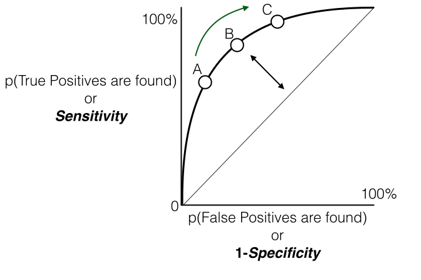Create three scenarios from ROC Curve

Scenario-1  (Point A on the ROC curve )

• Imagine that t1 is the threshold value which results in the point A. t1- gives some sensitivity and specificity.
• If we take t1 as threshold value we have the below scenario
• True positive 65% and False Positive 10%
• To capture nearly 65% of the good(target) we are making 10% mistakes
• Are you happy with loosing 35% here and making only 10% mistakes there.
• For example, you are dealing with loans. Where your target is finding bad customer in a loans portfolio. Out all the laon applications your model successfully identified 65% of the bad customers. In that process, it also wrongly classified 10% of good customers as bad customers.
• So finally scenario -1 ; with probability threshold t1 : we have two losses 35% of bad customers will be given loans and 10% of good customers will be rejected loans.

Scenario-2  (Point B on the ROC curve )

• Imagine that t2 is the threshold value which results in the point B.
• If we take t2 as threshold value we have  the below scenario
• True positive 80% and False Positive 30%
• To capture nearly 80% of the good(target) we are making 30% mistakes
• Are you happy with capturing 80% here and making only  30% mistakes there.
• In our loans example, Out all the loan applications, your model successfully identified 80% of the bad customers. In that process, it also wrongly classified 30% of good customers as bad customers.
• Now scenario -2 ; with probability threshold t2: we have two losses 20% of bad customers will be given loans and 30% of good customers will be rejected loans.

Scenario-3 (Point C on the ROC curve )

• Imagine that t3 is the threshold value which results in the point C.
• True positive 90% and False Positive 60%
• To capture nearly 90% of the good(target) we are making 60% mistakes
• Are you happy with capturing 90% here and making  as many as 60% mistakes there.
• In our loans example, Out all the loan applications, your model successfully identified 90% of the bad customers. In that process, it also wrongly classified 60% of good customers as bad customers.
• Now scenario -3 ; with probability threshold t3: we have two losses 10% of bad customers will be given loans and 60% of good customers will be rejected loans.

Scenario Analysis Conclusion:

• If the problem that you are handling is detecting a bomb, then you may want to be nearly 100% accurate, which means you will make lot of mistakes (False positives). Scenario-3
• In loans portfolio you don’t want to loose lot of good customers.  You would prefer scenario-1 or scenario-2.
• If it is e-mail marketing and you want to capture as many responders as possible then you will choose Scenario-3
• If its is telephone outbound call marketing then you don’t want to unnecessarily call non-responders.  There is a cost associated with false positives. You would prefer scenario-1 or scenario-2.

ROC and AUC

• We want that curve to be far away from straight line. Ideally we want the area under the curve as high as possible
• ROC comes with a connected topic, AUC. Area Under the Curve
• ROC Curve Gives us an idea on the performance of the model under all possible values of threshold.
• We want to make almost 0% mistakes while identifying all the positives, which means we want to see AUC value near to 1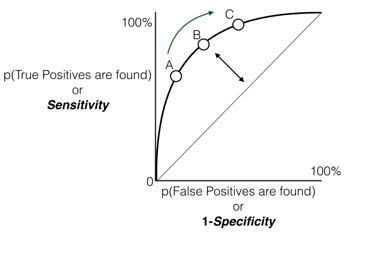AUC

• AUC is near to 1 for a good model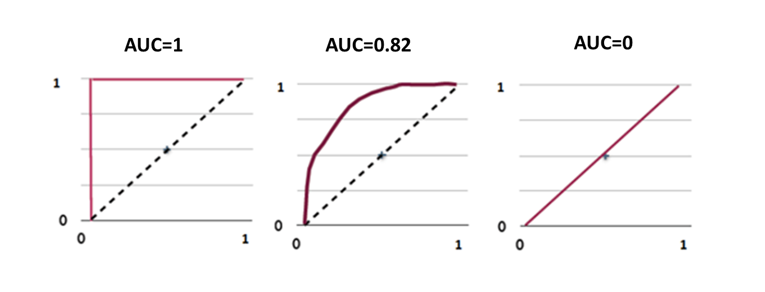ROC and AUC Calculation

Building a Logistic Regression Model

prod_sales_Logit_model<- glm(Bought ~ Age, family=binomial,data=Product_slaes)
summary(prod_sales_Logit_model)
##
## Call:
## glm(formula = Bought ~ Age, family = binomial, data = Product_slaes)
##
## Deviance Residuals:
##     Min       1Q   Median       3Q      Max
## -3.6922  -0.1645  -0.0619   0.1246   3.5378
##
## Coefficients:
##             Estimate Std. Error z value Pr(>|z|)
## (Intercept) -6.90975    0.72755  -9.497   <2e-16 ***
## Age          0.21786    0.02091  10.418   <2e-16 ***
## ---
## Signif. codes:  0 '***' 0.001 '**' 0.01 '*' 0.05 '.' 0.1 ' ' 1
##
## (Dispersion parameter for binomial family taken to be 1)
##
##     Null deviance: 640.425  on 466  degrees of freedom
## Residual deviance:  95.015  on 465  degrees of freedom
## AIC: 99.015
##
## Number of Fisher Scoring iterations: 7

Code – ROC Calculation

library(pROC)
## Warning: package 'pROC' was built under R version 3.1.3
## Type 'citation("pROC")' for a citation.
##
## Attaching package: 'pROC'
## The following objects are masked from 'package:stats':
##
##     cov, smooth, var
predicted_prob<-predict(prod_sales_Logit_model,type="response")
roccurve <- roc(prod_sales_Logit_model\$y, predicted_prob)
plot(roccurve)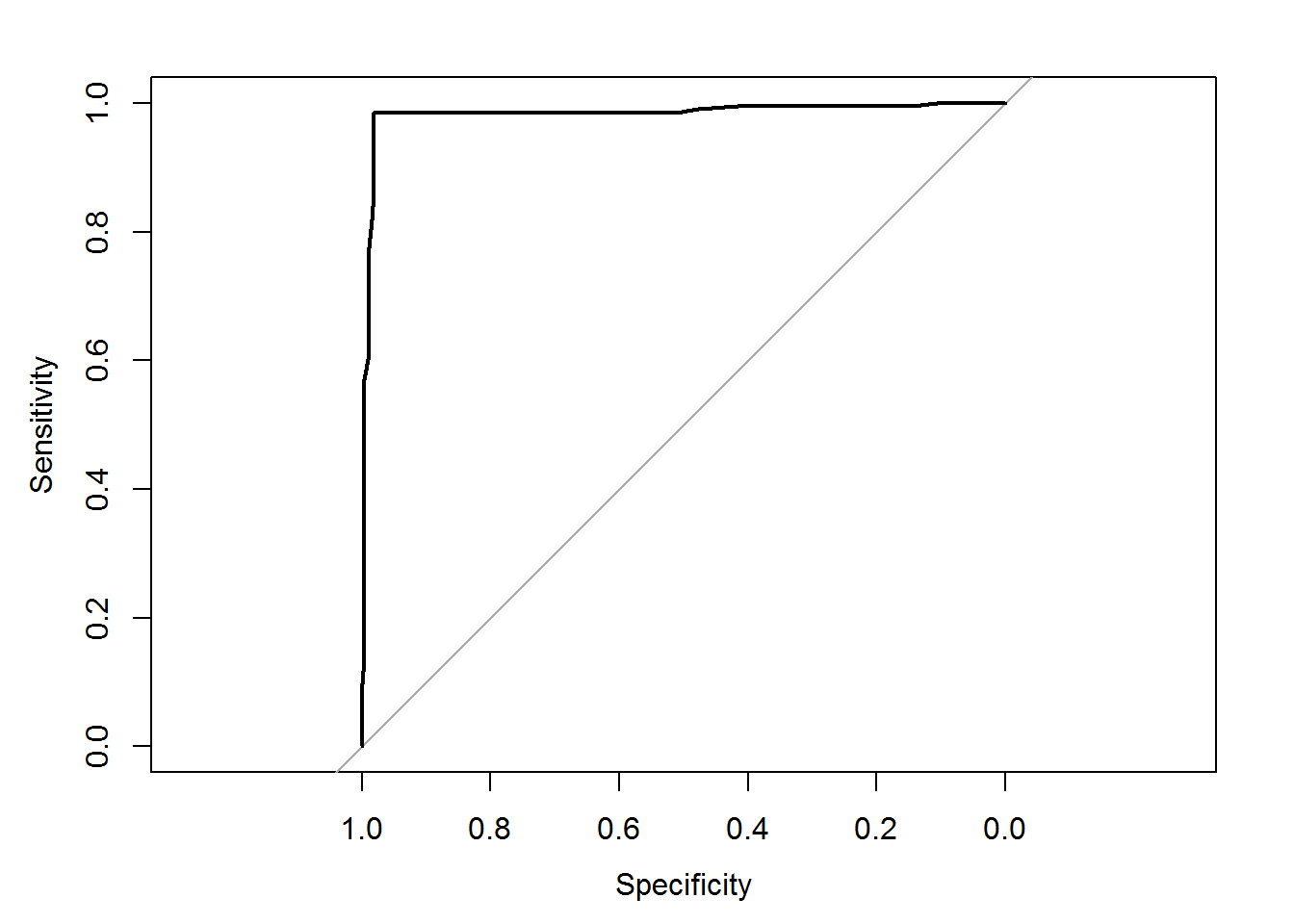##
## Call:
## roc.default(response = prod_sales_Logit_model\$y, predictor = predicted_prob)
##
## Data: predicted_prob in 262 controls (prod_sales_Logit_model\$y 0) < 205 cases (prod_sales_Logit_model\$y 1).
## Area under the curve: 0.983

Code – AUC Calculation

auc(roccurve)
## Area under the curve: 0.983

Or

auc(prod_sales_Logit_model\$y, predicted_prob)
## Area under the curve: 0.983

Code-ROC from Fiberbits Model

predicted_prob<-predict(Fiberbits_model_1,type="response")
roccurve <- roc(Fiberbits_model_1\$y, predicted_prob)
plot(roccurve)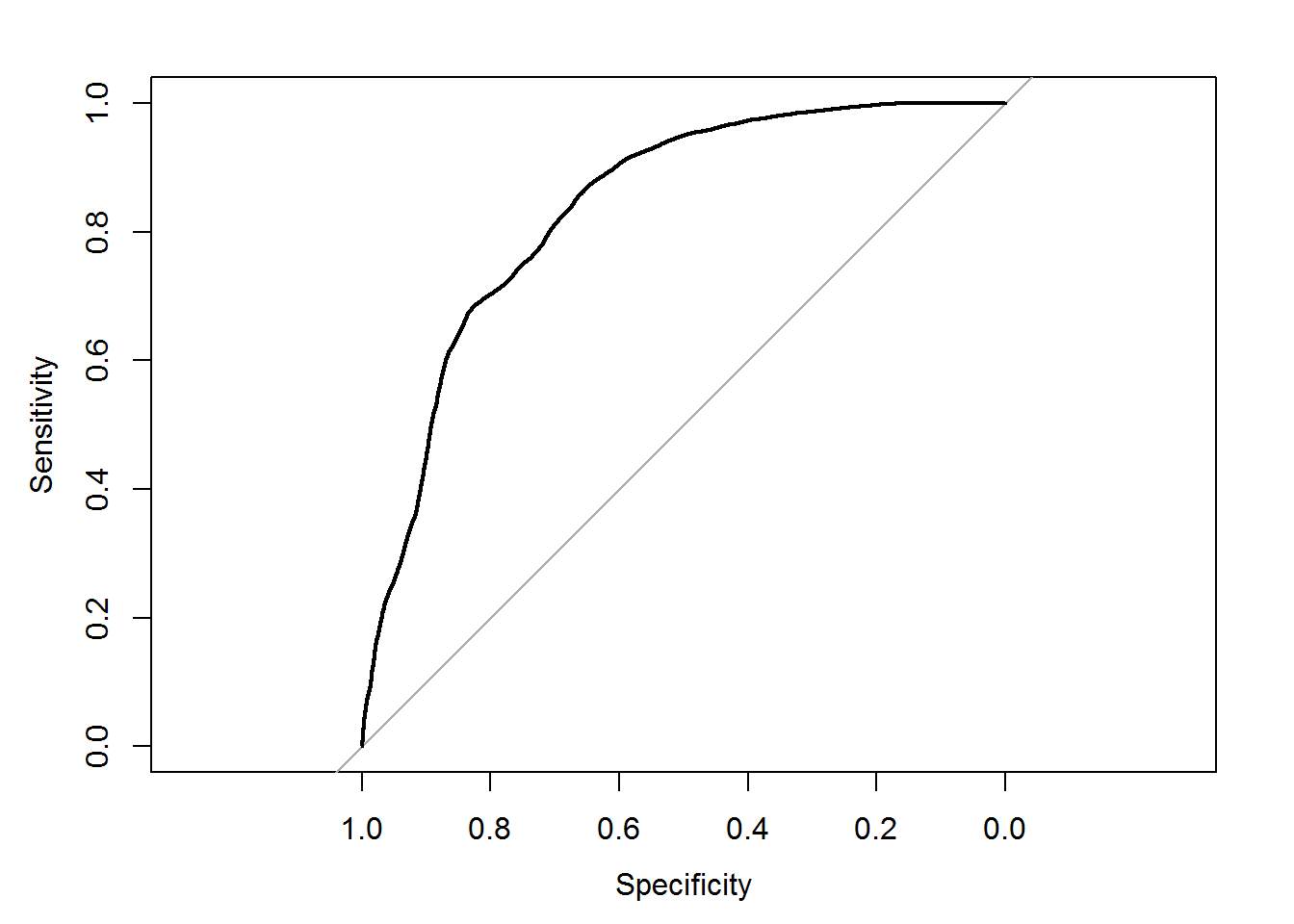##
## Call:
## roc.default(response = Fiberbits_model_1\$y, predictor = predicted_prob)
##
## Data: predicted_prob in 42141 controls (Fiberbits_model_1\$y 0) < 57859 cases (Fiberbits_model_1\$y 1).
## Area under the curve: 0.835

Code-AUC of Fiberbits Model

auc(roccurve)
## Area under the curve: 0.835

What is a best model? How to build?

• A model with maximum accuracy /least error
• A model that uses maximum information available in the given data
• A model that has minimum squared error
• A model that captures all the hidden patterns in the data
• A model that produces the best perdition results

The next post is about What is the Best Model.

24th January 2018
0 responses on "203.4.3 ROC and AUC"